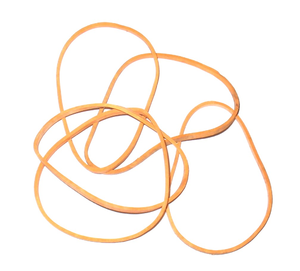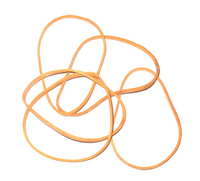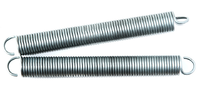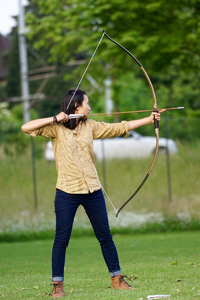# Elastic Potential Energy Store

## Key Stage 3

### MeaningRubber bands can store energy in their elastic potential store.

The elastic potential energy store is an energy store associated with stretched, squashed and twisted elastic objects.

The more an object is stretched the more energy it has in its elastic potential energy store.

### ExamplesRubber bands can store elastic potential energy when they are stretched. A spring stores energy in the elastic potential store when they are stretched, squashed and bent. A stretched bow stores elastic potential energy. The further it is stretched the more energy it stores.

## Key Stage 4

### Meaning

The elastic potential energy store is an energy store associated with stretched, squashed and twisted elastic objects.

Any object that returns to its original shape after a deforming force has been removed is able to store elastic potential energy.
Elastic potential energy is a Potential Energy store.
The elastic potential energy store of an object is related to two properties of the object:
• The Spring Constant of the object. - The amount of force needed to stretch or squash it's shape by a given distance. The greater the spring constant the greater the elastic potential energy stored.
• The extension of the object. - The distance that the object has been stretched or squashed. The greater the extension the greater the elastic potential energy stored.

### Equation

NB: You will be given this equation in the formula sheet.

Elastic Potential Energy = 0.5 x (Spring Constant) x (Extension)2

$$E_e = \frac{1}{2} k x^2$$

Where:

Ee = Elastic Potential Energy stored.

k = The spring constant of the object.

x = The extension of the object.

### Calculating Elastic Potential Energy

 A bow with a spring constant of 400N/m is stretched 0.5m with a force of 200N. Calculate the elastic potential store of the bow. A bungee cord with a spring constant of 45N/m stretches by 30m. Calculate the elastic potential store of the cord. Give your answer correct to 2 significant figures. A slinky spring of length 0.1m and spring constant 0.80N/m is stretched to a length of 9.1m. Calculate the elastic potential store of the slinky. Give your answer correct to 2 significant figures. 1. State the known quantities k = 400N/m x = 0.5m 1. State the known quantities k = 45N/m x = 30m 1. State the known quantities k = 0.8N/m Original Length = 0.1m Final Length = 9.1m Find the extension. x = Final Length - Original Length = 9.1 - 0.1 = 9.0m 2. Substitute the numbers into the equation and solve. $$E_e = \frac{1}{2} k x^2$$ $$E_e = \frac{1}{2} \times 400 \times 0.5^2$$ $$E_e = \frac{1}{2} \times 400 \times 0.25$$ $$E_e = 50J$$ 2. Substitute the numbers into the equation and solve. $$E_e = \frac{1}{2} k x^2$$ $$E_e = \frac{1}{2} \times 45 \times 30^2$$ $$E_e = \frac{1}{2} \times 45 \times 900$$ $$E_e = 20250J$$ $$E_e \approx 20,000J$$ 2. Substitute the numbers into the equation and solve. $$E_e = \frac{1}{2} k x^2$$ $$E_e = \frac{1}{2} \times 0.80 \times 9.0^2$$ $$E_e = \frac{1}{2} \times 0.80 \times 81$$ $$E_e = 32.4J$$ $$E_e \approx 32J$$